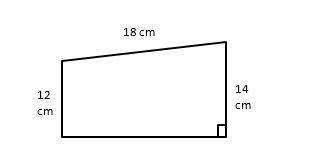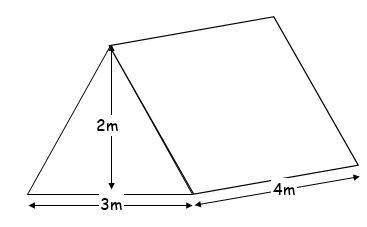# FREE 8th Grade Georgia Milestones Assessment System Math Practice TestWelcome to our FREE Georgia Milestones Assessment System Math practice test for 8th Grade, with answer key and answer explanations. This practice test’s realistic format and high-quality practice questions can help your student succeed on the Georgia Milestones Assessment System Math test. Not only does the test closely match what students will see on the real Georgia Milestones Assessment System, but it also comes with detailed answer explanations.

For this practice test, we’ve selected 20 real questions from past exams for your student’s Georgia Milestones Assessment System Practice test. Your student will have the chance to try out the most common Georgia Milestones Assessment System Math questions. For every question, there is an in-depth explanation of how to solve the question and how to avoid mistakes next time.

Use our free Georgia Milestones Assessment System Math practice tests and study resources (updated for 2021) to help your students ace the Georgia Milestones Assessment System Math test! Make sure to follow some of the related links at the bottom of this post to get a better idea of what kind of mathematics questions students need to practice.

## 10 Sample 8th Grade Georgia Milestones Assessment System Math Practice Questions

1- What is the slope of a line that is perpendicular to the line
$$4x-2y=12$$ ?

A. 2

B. 1

C. $$-2$$

D. $$-\frac{1}{2}$$

2- The diagonal of a rectangle is 10 inches long and the height of the rectangle is 8 inches. What is the perimeter of the rectangle in inches?

3- You can buy 5 cans of green beans at a supermarket for $3.40. How much does it cost to buy 35 cans of green beans? A.$17

B. $23.80 C.$34.00

D. $119 4- Which of the following is the solution of the following inequality? $$2x+4>11x-12.5-3.5x$$ A. $$x<3$$ B. $$x>3$$ C. $$x≤4$$ D. $$x≥4$$ 5- What is the perimeter of a square that has an area of 595.36 feet? 6- A tree 32 feet tall casts a shadow 12 feet long. Jack is 6 feet tall. How long is Jack’s shadow? A. 2.25 ft B. 4 ft C. 4.25 ft D. 8 ft 7- The perimeter of the trapezoid below is 54 cm. What is its area?8- Which graph does not represent $$y$$ as a function of $$x$$? A. B. C. D. 9- Which of the following is equivalent to $$13<-3x-2<22$$ ? A. $$-8 < x < -5$$ B. $$5 < x < 8$$ C. $$\frac{11}{3} < x < \frac{20}{3}$$ D. $$\frac{-20}{3} < x < \frac{-11}{3}$$ 10- In a certain bookshelf of a library, there are 35 biology books, 95 history books, and 80 language books. What is the ratio of the number of biology books to the total number of books in this bookshelf? A. $$\frac{1}{4}$$ B. $$\frac{1}{6}$$ C. $$\frac{2}{7}$$ D. $$\frac{3}{8}$$ 11- A bank is offering $$3.5\%$$ simple interest on a savings account. If you deposit$12,000, how much interest will you earn in two years?

A. $420 B.$840

C. $4200 D.$8400

12- The area of a circle is $$64 π$$. What is the circumference of the circle?

A. $$8 π$$

B. $$16 π$$

C. $$32 π$$

D. $$64 π$$

13- A shirt costing \$200 is discounted $$15\%$$. After a month, the shirt is discounted another $$15\%$$. Which of the following expressions can be used to find the selling price of the shirt?

A. $$(200) (0.70)$$

B. $$(200) – 200 (0.30)$$

C. $$(200) (0.15) – (200) (0.15)$$

D. $$(200) (0.85) (0.85)$$

14- Joe scored 20 out of 25 marks in Algebra, 30 out of 40 marks in science and 68 out of 80 marks in mathematics. In which subject his percentage of marks is best?

A. Algebra

B. Science

C. Mathematics

D. Algebra and Science

15- What is the volume of the following triangular prism?16- The marked price of a computer is D dollar. Its price decreased by $$20\%$$ in January and later increased by $$10\%$$ in February. What is the final price of the computer in D dollar?

A. 0.80 D

B. 0.88 D

C. 0.90 D

D. 1.20 D

17- Triangle ABC is graphed on a coordinate grid with vertices at A $$(–3, –2)$$, B $$(–1, 4)$$ and C $$(7, 9)$$. Triangle ABC is reflected over$$x$$ axes to create triangle A’ B’ C’.
Which order pair represents the coordinate of C’?

A. $$(7, 9)$$

B. $$(–7, –9)$$

C. $$(–7, 9)$$

D. $$(7, –9)$$

18-

What’s the maximum ratio of woman to man in the four cities?

A. 0.98

B. 0.97

C. 0.96

D. 0.95

19-

What’s the ratio of percentage of men in city A to percentage of women in city C?

A. 0.9

B. 0.95

C. 1

D. 1.05

20-

A container holds 3.5 gallons of water when it is $$\frac{7}{24}$$ full. How many gallons of water does the container hold when it’s full?

A. 8

B. 12

C. 16

D. 20

## Answers:

1- D
The equation of a line in slope intercept form is:$$y=mx+b$$
Solve for $$y$$.
$$4x-2y=12 {\Rightarrow} -2y=12-4x {\Rightarrow} y=(12-4x){\div}(-2) {\Rightarrow} y=2x-6$$
The slope of this line is 2.
The product of the slopes of two perpendicular lines is$$-1$$.
Therefore, the slope of a line that is perpendicular to this line is:
$$m_{1} {\times} m_{2} = -1 {\Rightarrow} 2 {\times} m_{2} = -1 {\Rightarrow} m_{2} = \frac{-1}{2}$$

2- 28

Let$$x$$ be the width of the rectangle. Use Pythagorean Theorem:
$$a^2 + b^2 = c^2$$
$$x^2 + 8^2 = 10^2 {\Rightarrow} x^2 + 64 = 100 {\Rightarrow} x^2 = 100 – 64 = 36 ⇒ x = 6$$
Perimeter of the rectangle =$$2 (length + width) = 2 (8 + 6) = 2 (14) = 28$$

3- B
Let $$x$$ be the number of cans. Write the proportion and solve for $$x$$.
$$\frac{5 \space cans}{ 3.40}=\frac{35 \space cans}{x}$$
$$x =\frac{3.40×35}{5}⇒x=23.8$$

4- A
$$2x+4>11x-12.5-3.5x$$→ Combine like terms:
$$2x+4>7.5x-12.5→$$ Subtract $$2x$$ from both sides: $$4>5.5x-12.5$$
Add 12.5 both sides of the inequality.
$$16.5>5.5x,$$
Divide both sides by 5.5.
$$\frac{16.5}{5}>x→x<3$$

5- 97.6
Area of a square: $$S = a^2 ⇒ 595.36 = a^2 ⇒ a = 24.4$$
Perimeter of a square: $$P = 4a ⇒ P = 4 × 24.4 ⇒ P = 97.6$$

6- A
Write the proportion and solve for the missing number.
$$\frac{32}{12}=\frac{6}{x}→ 32x=6×12=72$$
$$32x=72→x=\frac{72}{32}=2.25$$

7- 130
The perimeter of the trapezoid is 54 cm.
Therefore, the missing side (high) is $$54 – 18 – 12 – 14 = 10$$
Area of a trapezoid: $$A = \frac{1}{2} h (b_1 + b_2) = \frac{1}{2} (10) (12 + 14) = 130$$

8- C
A graph represents $$y$$ as a function of $$x$$ if
$$x_1=x_2→y_1=y_2$$
In choice C, for each $$x$$, we have two different values for $$y$$.

9- A
$$13<-3x-2<22$$→ Add 2 to all sides. $$13+2<-3x-2+2<22+2$$
$$→15<-3x<24$$→ Divide all sides by $$- 3$$. (Remember that when you divide all sides of an inequality by a negative number, the inequality sing will be swapped. < becomes >)
$$\frac{15}{-3} > \frac{-3x}{3} >\frac{24}{-3}$$
$$-8 < x < -5$$

10- B
Number of biology book: 35
Total number of books; $$35+95+80=210$$
The ratio of the number of biology books to the total number of books is: $$\frac{35}{210}=\frac{1}{6}$$

11- B
Use simple interest formula:
I=prt
(I = interest, p = principal, r = rate, t = time)
$$I=(12000)(0.035)(2)=840$$

12- B
Use the formula for area of circles.
Area $$= πr^2 ⇒ 64 π = πr^2 ⇒ 64 = r^2 ⇒ r = 8$$
Radius of the circle is 8. Now, use the circumference formula:
Circumference$$= 2πr = 2π (8) = 16 π$$

13- D
To find the discount, multiply the number by ($$100\% –$$ rate of discount).
Therefore, for the first discount we get: $$(200) (100\% – 15\%) = (200) (0.85)$$
For the next $$15\%$$ discount: $$(200) (0.85) (0.85)$$

14- C
Compare each mark:
In Algebra Joe scored 20 out of 25 in Algebra. It means Joe scored $$80\%$$ of the total mark.
$$\frac{20}{25}=\frac{x}{100}⇒x= 80%$$
Joe scored 30 out of 40 in science. It means Joe scored $$75\%$$ of the total mark.
$$\frac{30}{40}=\frac{x}{100}⇒x= 75%$$
Joe scored 68 out of 80 in mathematic that it means $$85\%$$ of total mark.
$$\frac{68}{80}=\frac{x}{100}⇒x= 85%$$
Therefore, his score in mathematic is higher than his other scores.

15- 12
Use the volume of the triangular prism formula.
$$V =\frac{1}{2} (length) (base) (high)$$
$$V = \frac{1}{2} × 4 × 3 × 2 ⇒ V = 12 \space m^3$$

16- B
To find the discount, multiply the price by ($$100\% –$$ rate of discount).
Therefore, for the first discount we get: $$(D) (100\% – 20\%) = (D) (0.80) = 0.80 D$$
To increase the $$10 \%: (0.80 D) (100\% + 10\%) = (0.85 D) (1.10) = 0.88 D = 88\%$$ of $$D$$

17- D
When a point is reflected over $$x$$ axes, the $$(y)$$ coordinate of that point changes to $$(-y)$$ while its $$x$$ coordinate remains the same.
$$C (7, 9) → C’ (7, -9)$$

18- B
Ratio of women to men in city A: $$\frac{570}{600}=0.95$$
Ratio of women to men in city B: $$\frac{291}{300}=0.97$$
Ratio of women to men in city C: $$\frac{665}{700}=0.95$$
Ratio of women to men in city D: $$\frac{528}{550}=0.96$$

19- D
Percentage of men in city $$A = \frac{600}{1170}×100=51.28%$$
Percentage of women in city $$C = \frac{665}{1365}×100=48.72%$$
Percentage of men in city $$A$$ to percentage of women in city $$C =\frac{51.28}{48.72}=1.05$$

20- B
let $$x$$ be the number of gallons of water the container holds when it is full.
Then;$$\frac{7}{24}x=3.5→x=\frac{24×3.5}{7}=12$$

Looking for the best resource to help you succeed on the Grade 8 Georgia Milestones Assessment System Math test?

### The Best Books to Ace 8th Grade Georgia Milestones Assessment SystemMath Test30% OFF

X

## How Does It Work?### 1. Find eBooks

Locate the eBook you wish to purchase by searching for the test or title.### 2. Add to Cart

Add the eBook to your cart.### 3. Checkout

Complete the quick and easy checkout process.### 4. Download

Immediately receive the download link and get the eBook in PDF format.

## Why Buy eBook From Effortlessmath?Save up to 70% compared to printInstantly download and access your eBookHelp save the environmentLifetime access to your eBookOver 2,000 Test Prep titles availableOver 80,000 happy customersOver 10,000 reviews with an average rating of 4.5 out of 524/7 supportAnytime, Anywhere Access